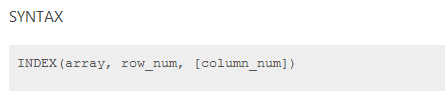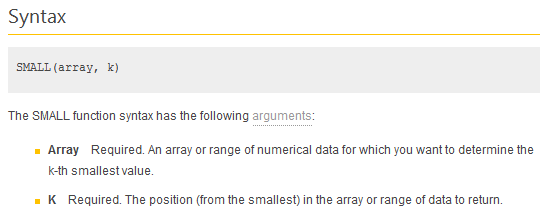# Formula Forensics 018. Retrieving the Nth number from a Range which has Gaps.

Over Easter, while we were all busy eating our Easter Eggs, over at the Chandoo.org/Forums, Slk213 was worried about how to retrieve the Nth number from a row of data which had gaps in it.

I am trying to pull the Nth number in a range of numbers.

I have a range of scores from G3 to L3. I am trying to create a formula in cells B3 thru F3. In cell B3, I am looking for the 1st score in range G3 thru L3 excluding blank spaces which would be 45. In C3 I am looking for the 2nd number in range G3 thru L3 excluding blanks spaces which would be 44. This would continue thru finding the 5th number.

Luckily I had finished my easter eggs and dropped a quick answer in for Slk213.

=INDEX(\$A\$3:\$L\$3,,SMALL(IF(\$G3:\$L3<>””,COLUMN(\$G3:\$L3)),COLUMN()-COLUMN(\$A\$1))) Ctrl Shift Enter

So today on Formula Forensics we will look at how this formula jumps over gaps in a range to retrieve the next item from the range, as quick as you can finish your Easter Egg.

## Retrieving the Nth Number.

Todays formula to retrieve the Nth Number from a list with gaps is:

=INDEX(\$A\$3:\$L\$3,,SMALL(IF(\$G3:\$L3<>””,COLUMN(\$G3:\$L3)),COLUMN()-COLUMN(\$A\$1))) Ctrl Shift Enter

We know that Index looks up a Range/Array and retrieves a value from a position in that Range/Array

The Index function has the syntax:In our formula:

=INDEX(\$A\$3:\$L\$3,,SMALL(IF(\$G3:\$L3<>””,COLUMN(\$G3:\$L3)),COLUMN()-COLUMN(\$A\$1)))

Array:              \$A\$3:\$L\$3

Row_Num: Nul or Blank = Same Row

Column_Num: SMALL(IF(\$G3:\$L3<>””,COLUMN(\$G3:\$L3)),COLUMN()-COLUMN(\$A\$1))

We can see from above that the Array is a single Row, Row 3, from A3 to L3

And we can see that the Index function Is looking up in the same row as the Row_Num is blank.

So all the work occurs in the Column_Num field of the Index function.

Lets have a look at the Column_Num function:

SMALL(IF(\$G3:\$L3<>””,COLUMN(\$G3:\$L3)),COLUMN()-COLUMN(\$A\$1))

We can see that it is made up of a Small Function

SMALL(IF(\$G3:\$L3<>””,COLUMN(\$G3:\$L3)),COLUMN()-COLUMN(\$A\$1))

The Small function has the syntaxIn our example

ArrayIF(\$G3:\$L3<>””,COLUMN(\$G3:\$L3))

k:    COLUMN()-COLUMN(\$A\$1)

Lets look at the array function IF(\$G3:\$L3<>””,COLUMN(\$G3:\$L3))

In a spare cell B14 enter = IF(\$G3:\$L3<>””,COLUMN(\$G3:\$L3)) press F9 not Enter

Excel responds with ={7,8,9,FALSE,11,12}

This is the Array answer to the formula IF(\$G3:\$L3<>””,COLUMN(\$G3:\$L3))

If the value in the Range G3:L3 is not Blank, return the value of the True component of the If. In this case it is the formula =COLUMN(\$G3:\$L3).

As this is an array formula It will return the 1st value from the True statement COLUMN(\$G3:\$L3) for the first value of the If Function.

It will return the 2nd value from the True statement COLUMN(\$G3:\$L3) for the 2nd value of the If Function. etc

If the value in the Range G3:L3 is Blank, return the value of the False component of the If, which is Blank and so If will return False

From the Excel response ={7,8,9,FALSE,11,12}

We can see that the 1st, 2nd, 3rd, 5th and 6th values are not Blank and so the Column No is returned ie: 7,8,9,11 & 12.

The 4th value in G3:L3 is Blank and so Excel has returned False, as J3=”” (Blank)

If we jump back to the Small function

SMALL(IF(\$G3:\$L3<>””,COLUMN(\$G3:\$L3)),COLUMN()-COLUMN(\$A\$1))

and substitute the array for the If function

The small function is now

SMALL({7,8,9,FALSE,11,12},COLUMN()-COLUMN(\$A\$1))

The second component of the Small function is k

k is the location in the array that you want returned.

In this example k = COLUMN()-COLUMN(\$A\$1)

This is the same as saying

k = Current Column – Column A1

k = Current Column – 1

We need to note that the location of the formula is important as it is measuring the offset using the position of the formula compared to Column A.

Slk213 wanted the formula in B3:F3

So the first cell B3 will return the value of

k = COLUMN()-COLUMN(\$A\$1)

k = COLUMN(B3)-COLUMN(A1)

k = 2 – 1

k = 1

So in Cell B3 the Small function will return the smallest value from the array, which is the lowest column Number or 7

In Cell C3 the Small function will return the 2nd smallest value from the array, which is the 2nd lowest column Number of 8

In Cell D3 the Small function will return the 3rd smallest value from the array, which is the lowest column Number or 9

In Cell E3 the Small function will return the 4th smallest value from the array, which is the 4th lowest column Number of 11

Note: By going from D3 to E3 we have skipped over the Blank cell which has a value in the array of False

We can now look at this Small component

In a spare cell B16 enter

=SMALL(IF(\$G3:\$L3<>””,COLUMN(\$G3:\$L3)),COLUMN()-COLUMN(\$A\$1)) Press F9 not Enter,

Excel will respond with a ={7}

Copy the formula to the adjacent cells C16:E16 and evaluate each with F9

Now in B18 try the following

=SMALL({7,8,9,FALSE,11,12},COLUMN()-COLUMN(\$A\$1))

Press F9 not Enter, Excel will respond with a ={7}

Copy the formula to the adjacent cells C18:E18 and evaluate each with F9

Because the formula =SMALL(IF(\$G3:\$L3<>””,COLUMN(\$G3:\$L3)),COLUMN()-COLUMN(\$A\$1)) is returning an array as an answer and the array answer is dependent on its location on the worksheet.

We can now use this as the lookup value from the original Index formula.

=INDEX(\$A\$3:\$L\$3,,SMALL(IF(\$G3:\$L3<>””,COLUMN(\$G3:\$L3)),COLUMN()-COLUMN(\$A\$1)))

Remembering that SMALL(IF(\$G3:\$L3<>””,COLUMN(\$G3:\$L3)),COLUMN()-COLUMN(\$A\$1)) is dependent on the position of the formula we can substitute the array answers for the Small function

That is in B3:

=INDEX(\$A\$3:\$L\$3,,SMALL(IF(\$G3:\$L3<>””,COLUMN(\$G3:\$L3)),COLUMN()-COLUMN(\$A\$1)))

=INDEX(\$A\$3:\$L\$3,,{7})

So Index will return the 7th value from the Range A3:L3, which is G3 or 45

In D3:

=INDEX(\$A\$3:\$L\$3,,SMALL(IF(\$G3:\$L3<>””,COLUMN(\$G3:\$L3)),COLUMN()-COLUMN(\$A\$1)))

=INDEX(\$A\$3:\$L\$3,,{9})

So Index will return the 9th value from the Range A3:L3, which is I3 or 43

In E3:

=INDEX(\$A\$3:\$L\$3,,SMALL(IF(\$G3:\$L3<>””,COLUMN(\$G3:\$L3)),COLUMN()-COLUMN(\$A\$1)))

=INDEX(\$A\$3:\$L\$3,,{11})

So Index will return the 11th value from the Range A3:L3, which is K3 or 42

Effectively skipping over the blank cells.

## Formula Forensics “The Series”

Formula Forensic Series

## Formula Forensics Needs Your Help

I urgently need more ideas for future Formula Forensics posts and so I need your help.

If you have a neat formula that you would like to share and explain, try putting pen to paper and draft up a Post like above or;

If you have a formula that you would like explained but don’t want to write a post also send it to Chandoo or Hui.Hello Awesome...

My name is Chandoo. Thanks for dropping by. My mission is to make you awesome in Excel & your work. I live in Wellington, New Zealand. When I am not F9ing my formulas, I cycle, cook or play lego with my kids. Know more about me.

Thank you and see you around.

### Related articles:

 Written by Hui... Tags: column(), downloads, if(), INDEX(), Learn Excel, Microsoft Excel Formulas, small Home: Chandoo.org Main Page ? Doubt: Ask an Excel Question

### 6 Responses to “Formula Forensics 018. Retrieving the Nth number from a Range which has Gaps.”

1.Andrew says:

Great Job, I like it!

These posts help so much because you can use the formulas in other applications because we fully understand what they do now!

•Hui... says:

Thanx Andrew

2.KELSO says:

This is amazing... I searched for this solution for an hour... I have a much better understanding of all the involved functions. Thanks for posting!

3.Ufoo says:

Someone help please. I have understood the formula, but I have a slight problem with the true section of the if (COLUMN(\$G3:\$L3)). My problem is how and why to get number 1 for the the first row or column. I thought it is a must to get number 1 in the array (i.e. you should have COLUMN(\$G3:\$L3)-COLUMN(\$G&3)+1) but in most cases this is not done in Chandoo.org. I am a bit confused. Thanks

•Hui... says:

@Ufoo

The section COLUMN(\$G3:\$L3) simply makes an array of Column numbers for the data = {7,8,9,10,11,12}

The magic happens when some of these values are equal to False and so are skipped

So the small uses value of COLUMN()-COLUMN(\$A\$1) which changes for each position to extract the next non-blank cell from the Columns Numbered 7-12

Work through each cell in Rows 14 to 20 and follow the logic of the cells
Notice How Column 10 (J) isn't selected
When a Column has a blank, it gets skipped as it is False
specifically between D18 and E18

4.Ufoo says:

Thanks a lot for clarification

 « Creating Cash Flow Statement by Indirect Method – I Last chance to sign-up for my Australian Excel Masterclass [Reminder] »

### Get FREE Excel & Power-BI Newsletter

One email per week with Excel and Power BI goodness. Join 100,000+ others and get it free.